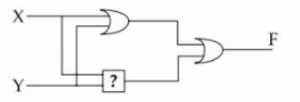## UGC NET CS 2005 Dec-Paper-2

 Question 1
T is a graph with n vertices. T is connected and has exactly n-1 edges, then :
 A T is a tree B T contains no cycles C Every pairs of vertices in T is connected by exactly one path D All of these
Engineering-Mathematics       Graph-Theory
Question 1 Explanation:
This is little bit tricky question.
Step-1:
n= number of vertices
n-1 = number of edges
Example: n=5 vertices and n-1=4 edgesStep-2: The above graph T won’t have cycle then we are calling as tree. Here, every pairs of vertices in T is connected by exactly one path.
Note: The above properties is nothing but minimum spanning tree properties.
 Question 2
If the proposition ​ ¬ ​ P ​ → ​ Q is true, then the truth value of the proportion ​ ¬ ​ PV (P ​ → ​ Q) is:
 A True B Multi - Valued C Flase D Can not determined
Engineering-Mathematics       Propositional-Logic
Question 2 Explanation:
We can also write (¬p → q) into (p ∨ q)Here, we can minimize the boolean form into
¬p ∨ (p → q)
= ¬p ∨ (¬p ∨ q)
= ¬p ∨ q
In question, they are not discussed about the truth values of p, it implies that ¬p ∨ q also be True or False. So, we cannot be determined.
 Question 3
Let A and B be two arbitrary events, then :
 A P(A∩B) = P(A) P(B) B P(P∪B) = P(A) + P(B) C P(A∪B) ≤ P(A) + P(B) D P(A​ / ​ B) = P(A∩B) + P(B)
Engineering-Mathematics       Probability
Question 3 Explanation:
Option-A is happens when A and B are independent.
Option-B is happens when A and B are mutually exclusive.
Option-C is not happens.
Option-D is P(A∪B) ≤ P(A) + P(B) is true because P(A∪B) = P(A) + P(B) - P(A∩B).
 Question 4
Which sentence can be generated by
S→d/bA
A→d/ccA
 A bccddd B aabccd C ababccd D abbbd E None of the above
Theory-of-Computation       Languages-and-Grammars
Question 4 Explanation:
Here, there is no terminal symbol ‘a’, so the answer never be option B,C,D.
Option-A is also wrong because the string generated by grammar is bccccd.Question 5
Regular expression a+b denotes the set :
 A {a} B {ε, a, b} C {a, b} D None of these
Theory-of-Computation       Regular-Expression
Question 5 Explanation:
The regular expression a+b denotes the set {a, b}.
 Question 6
Which of the following is divisible by 4 ?
 A 100101100 B 1110001110001 C 11110011 D 10101010101010
Digital-Logic-Design       Number-Systems
Question 6 Explanation:
Option-A: (100101100)​ 2​ = (300)​ 10
300 is divisible by 4
Option-B: (1110001110001)​ 2​ = (7281)​ 10
7281 is not divisible by 4
Option-C: (11110011)​ 2​ = (243)​ 10
243 is not divisible by 4
Option-D: (10101010101010)​ 2​ = (10,922)​ 10
10,922 is not divisible by 4.
 Question 7
A half-adder is also known as :
 A AND Circuit B NAND Circuit C NOR Circuit D EX-OR Circuit
Digital-Logic-Design       Combinational-Circuit
Question 7 Explanation:
→ The half adder adds two single binary digits A and B. It has two outputs, sum (S) and carry (C). The carry signal represents an overflow into the next digit of a multi-digit addition. The value of the sum is 2C + S. The simplest half-adder design incorporates an XOR gate for S and an AND gate for C. The Boolean logic for the sum (in this case S) will be A′B + AB′ whereas for the carry (C) will be AB.Question 8
Consider the following sequence of instructions :
a=a⊕b, b=a⊕b, a=b⊕a
This Sequence
 A retains the value of the a and b B complements the value of a and b C swap a and b D negates values of a and b
Digital-Logic-Design       Boolean-Expression
Question 8 Explanation:
⇒ a=a⊕b
⇒ b=a ⊕b
= a⊕b ⊕b (Substitute (1))
= 0 ⊕ b
= b
⇒ a = b⊕a
= b⊕a⊕b (Substitute (1))
= a⊕b⊕b
= a⊕0
= a
 Question 9
Consider the following circuit :to make it a Tautology the ​ ? ​ should be :
 A NAND gate B AND gate C OR gate D EX-OR gate
Digital-Logic-Design       Logic-Gates
Question 9 Explanation:
Method 1:
(X+Y) + (XY)’
= X+Y+X’+Y’
= (X+X’)+(Y+Y’)
= 1
Method 2:Question 10
When an inventor is placed between both inputs of an S-R flip flop, the resulting flip flop is :
 A JK flip-flop B D-flip-flop C T flip-flop D None of these
Digital-Logic-Design       Sequential-Circuits
Question 10 Explanation:
Given question is ambiguous. It is not mentioned how the inverter is connected. Placing NOT gate in different positions gives different solutions.
 Question 11
What is the output of the following C-program
main()
{
printf(''%d%d%d'', sizeof(3.14f), sizeof(3.14), sizeof(3.141));
}
 A 4 4 4 B 4 8 10 C 8 4 8 D 8 8 8 E None of the above
Programming       Operator
Question 11 Explanation:
The sizeof operator will print number of bytes of a data type.
sizeof(3.14f) → It will consider as float data type. The float data type size is 4 bytes.
sizeof(3.14) → It will consider as double data type. The double data type size is 8 bytes.
sizeof(3.141) → It will consider as double data type. The double data type size is 8 bytes.
Output= 4 8 8
Note: The exact size of each of these 3 types depends on the C compiler implementation (or) platform
 Question 12
The bitwise OR of 35 with 7 in C will be :
 A 35 B 7 C 42 D 39
Programming       Operator
Question 12 Explanation:
Step-1: First we have to convert into binary numbers
(35)​ 10​ = (​ 0010 0011​ ) ​ 2
(7)​ 10​ = (0000 0111)​ 2
Step-2: Perform OR operation on both binary numbers.
0010 0011
0000 0111
---------------
0010 0111
----------------
Step-3: Convert result into Decimal number
(0010 0111)​ 2​ = (39)​ 10
There are 12 questions to complete.

Register Now